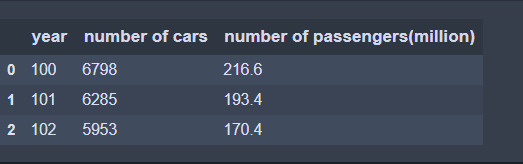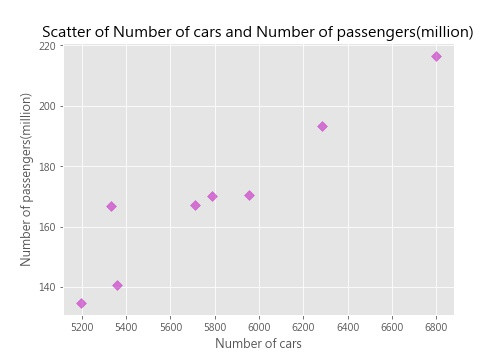#3

## [Python]資料視覺化M01─運用matplotlib完成散布圖(scatter)

Hi! 大家好，我是Eric，從這篇開始將是Python的系列教學文，會先從簡單的資料視覺化開始，後續可能會練習一些機器學習(machine learn)，請大家拭目以待@@• 緣起：台灣的公路客運路線越來越多，營運的車輛也跟著增加，那車輛數與載客數之間的關係為何呢?是否真的呈現正相關呢，就讓我們由資料中探索看看吧。
• 方法：運用 [Python]的[matplotlib] 套件。
• 使用資料：交通部公路總局統計查詢網-公路汽車客運業營運概況，100-107年
• 參考來源：台灣資料科學年會-手把手打開Python資料分析大門 https://www.slideshare.net/tw_dsconf/python-83977705

1. 載入套件。

``````import pandas as pd               # 資料處理套件
import matplotlib.pyplot as plt   # 資料視覺化套件
``````

2. 載入資料。

``````bus = pd.read_csv("bus.csv")
``````3. 開始畫圖。

``````plt.figure(figsize=(7,5))   # 顯示圖框架大小

plt.style.use("ggplot")     # 使用ggplot主題樣式
plt.xlabel("Number of cars", fontweight = "bold")                  #設定x座標標題及粗體
plt.ylabel("Number of passengers(million)", fontweight = "bold")   #設定y座標標題及粗體
plt.title("Scatter of Number of cars and Number of passengers(million)",
fontsize = 15, fontweight = "bold")        #設定標題、字大小及粗體

plt.scatter(bus["number of cars"],                    # x軸資料
bus["number of passengers(million)"],     # y軸資料
c = "m",                                  # 點顏色
s = 50,                                   # 點大小
alpha = .5,                               # 透明度
marker = "D")                             # 點樣式

plt.savefig("Scatter of Number of cars and Number of passengers(million).jpg")   #儲存圖檔
plt.close()      # 關閉圖表
``````

4. 大功告成。P.S. 本篇程式碼為參考台灣資料科學年會-手把手打開Python資料分析大門，並利用網路實際的開放資料執行